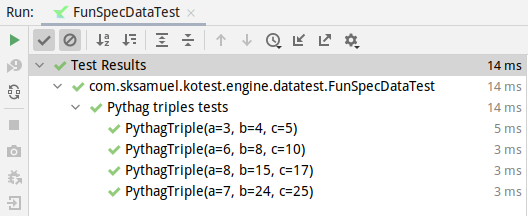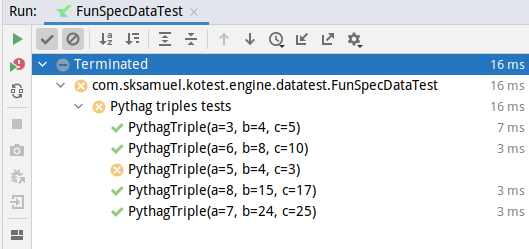Version: 5.2

# Introduction

##### Required Module

Before data-driven-testing can be used, you need to add the module `kotest-framework-datatest` to your build.

##### note

This section covers the new and improved data driven testing support that was released with Kotest 4.6.0. To view the documentation for the previous data test support, click here

When writing tests that are logic based, one or two specific code paths that work through particular scenarios make sense. Other times we have tests that are more example based, and it would be helpful to test many combinations of parameters.

In these situations, data driven testing (also called table driven testing) is an easy technique to avoid tedious boilerplate.

Kotest has first class support for data driven testing built into the framework. This means Kotest will automatically generate test case entries, based on input values provided by you.

## Getting Started​

Let's consider writing tests for a pythagorean triple function that returns true if the input values are valid triples (a squared + b squared = c squared).

``fun isPythagTriple(a: Int, b: Int, c: Int): Boolean = a * a + b * b == c * c``

Since we need more than one element per row (we need 3), we start by defining a data class that will hold a single row of values (in our case, the two inputs, and the expected result).

``data class PythagTriple(val a: Int, val b: Int, val c: Int)``

We will create tests by using instances of this data class, passing them into the `withData` function, which also accepts a lambda that performs the test logic for that given row.

For example:

``class MyTests : FunSpec({  context("Pythag triples tests") {    withData(      PythagTriple(3, 4, 5),      PythagTriple(6, 8, 10),      PythagTriple(8, 15, 17),      PythagTriple(7, 24, 25)    ) { (a, b, c) ->      isPythagTriple(a, b, c) shouldBe true    }  }})``

Notice that because we are using data classes, the input row can be destructured into the member properties. When this is executed, we will have 4 test cases in our input, one for each input row.

Kotest will automatically generate a test case for each input row, as if you had manually written a separate test case for each.The test names are generated from the data classes themselves but can be customized.

If there is an error for any particular input row, then the test will fail and Kotest will output the values that failed. For example, if we change the previous example to include the row `PythagTriple(5, 4, 3)` then that test will be marked as a failure.The error message will contain the error and the input row details:

`Test failed for (a, 5), (b, 4), (c, 3) expected:<9> but was:<41>`

In that previous example, we wrapped the `withData` call in a parent test, so we have more context when the test results appear. The syntax varies depending on the spec style used - here we used fun spec which uses context blocks for containers. In fact, data tests can be nested inside any number of containers.

But this is optional, you can define data tests at the root level as well.

For example:

``class MyTests : FunSpec({  withData(    PythagTriple(3, 4, 5),    PythagTriple(6, 8, 10),    PythagTriple(8, 15, 17),    PythagTriple(7, 24, 25)  ) { (a, b, c) ->    isPythagTriple(a, b, c) shouldBe true  }})``
##### caution

Data tests can only be defined at the root or in container scopes. They cannot be defined inside leaf scopes.